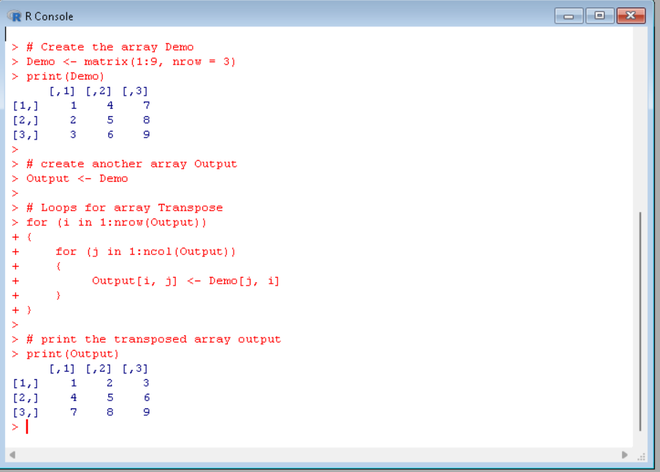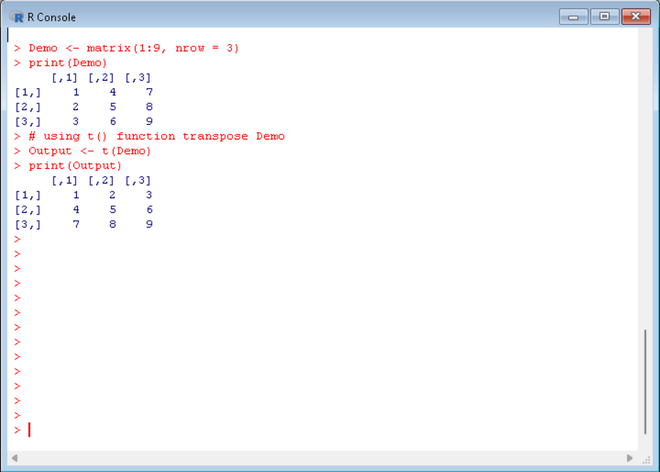# Array Transposition in R

• Last Updated : 14 Sep, 2021

In this article, we will discuss how can we transpose an array in the R programming language. The transpose of an array is obtained by changing rows to columns and columns to rows.

Aij = Aji

where i is not equal to j, thus diagonals remain unchanged.

Example:

```Array demo[] --->  [1, 4, 7
2, 5, 8,
3, 6, 9]
Transpose of demo[]:
Output --->   [1, 2, 3
4, 5, 6
7, 8, 9]```

There are two methods to get transpose of an array in R:

## Method 1: Naive approach

We can iterate over the array and assign the correspondent elements from row to column and column to row.

Example: Transpose of an array

## R

 `# Create the array Demo ` `Demo <- ``matrix``(1:9, nrow = 3) ` `print``(Demo) ` `   `  `# create another array Output ` `Output <- Demo ` `   `  `# Loops for array Transpose ` `for ``(i ``in` `1:``nrow``(Output)) ` `{    ` `    ``for ``(j ``in` `1:``ncol``(Output)) ` `    ``{  ` `         ``Output[i, j] <- Demo[j, i]  ` `    ``} ` `} ` `   `  `# print the transposed array output ` `print``(Output)`

Output:## Method 2: Using t() function

We can transpose an array directly in R using the inbuilt function t(). This function takes the array as a parameter and returns its transpose.

Syntax:

t(array)

Example: Transpose of an array

## R

 `# Create demo array ` `Demo <- ``matrix``(1:9, nrow = 3)  ` `print``(Demo) ` ` `  `# using t() function transpose Demo ` `Output <- ``t``(Demo)  ` `print``(Output)`

Output:My Personal Notes arrow_drop_up
Recommended Articles
Page :